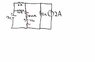# Norton's Equivalent Circuit

• Engineering
• johnsy1312

#### johnsy1312

Finding the Norton Equivalent circuit for the circuit attached

I attempted this solution but i am unsure if my Norton's current is correct:

$R_N = \frac{1}{\frac{1}{6}+\frac{1}{12}+\frac{1}{12}}=3ohms$

$I'_N = \frac{12*2}{12+4}=1.5A, I''_N = 2A$

$I_N = 2A - 1.5A = 0.5A$

#### Attachments

•tre.jpg
11.6 KB · Views: 391

Finding the Norton Equivalent circuit for the circuit attached

I attempted this solution but i am unsure if my Norton's current is correct:

$R_N = \frac{1}{\frac{1}{6}+\frac{1}{12}+\frac{1}{12}}=3ohms$

$I'_N = \frac{12*2}{12+4}=1.5A, I''_N = 2A$

$I_N = 2A - 1.5A = 0.5A$

Can you explain the logic behind your Norton current calculations? In particular, what motivates your calculation of ##I'_N## ?